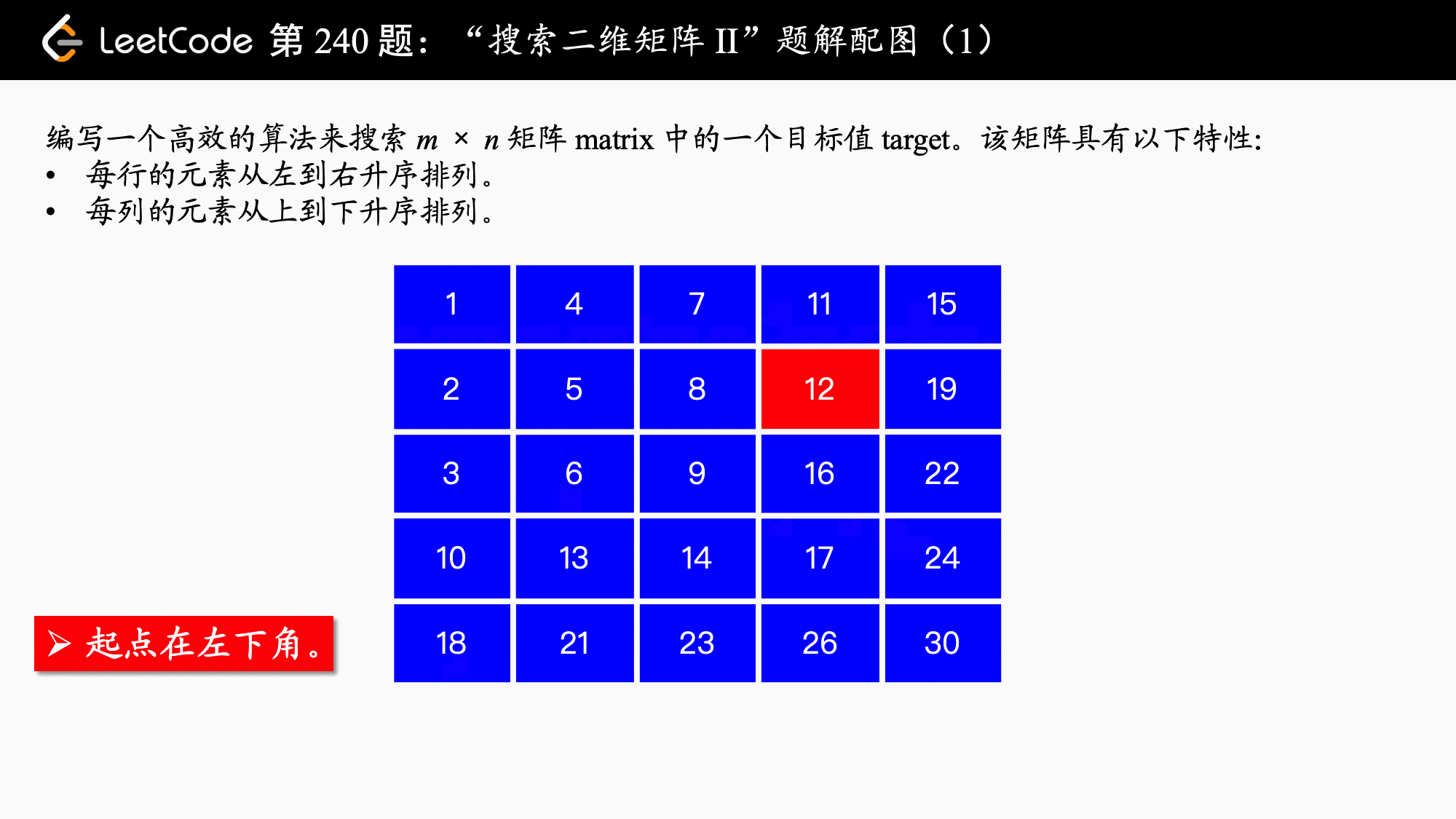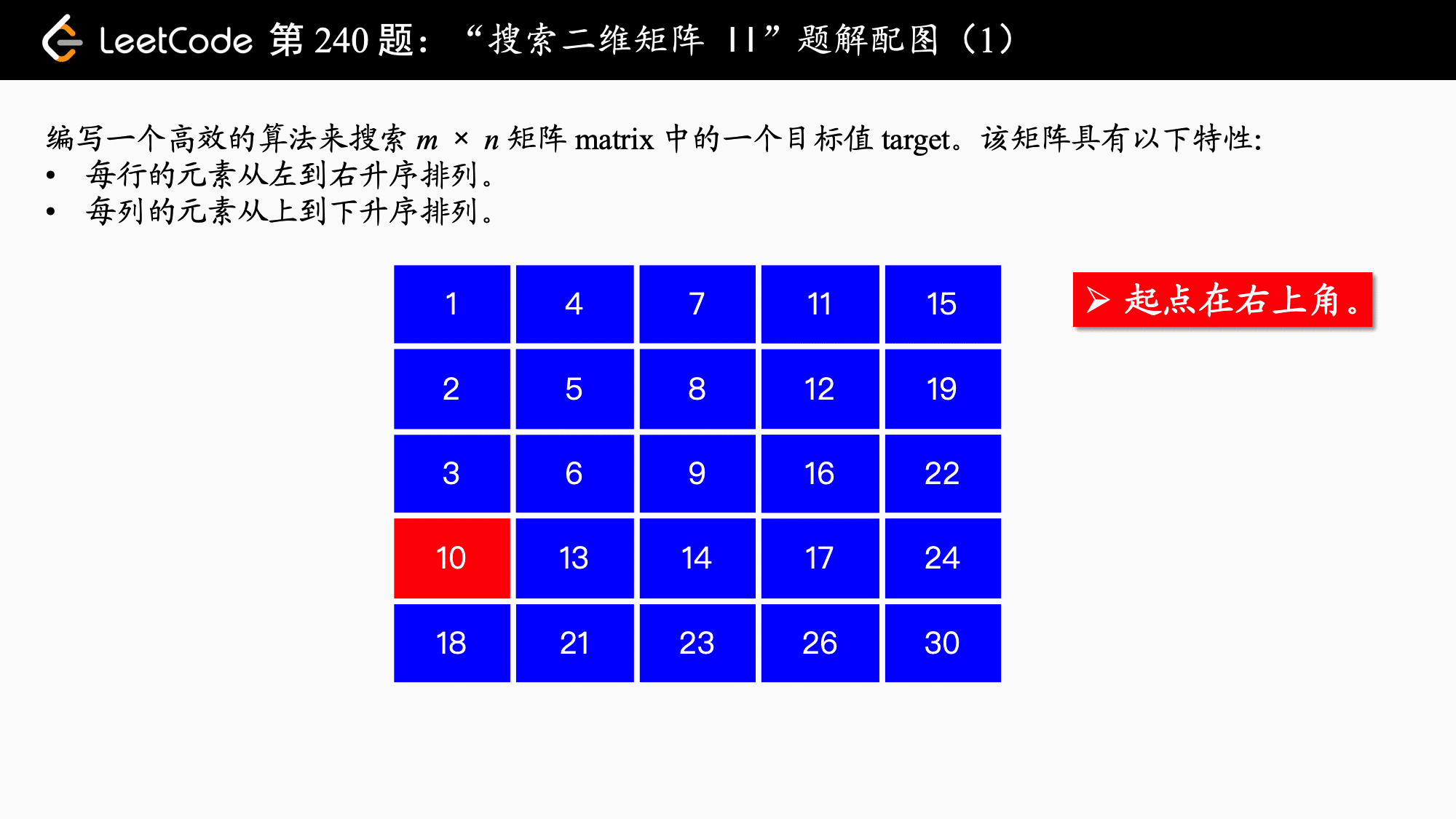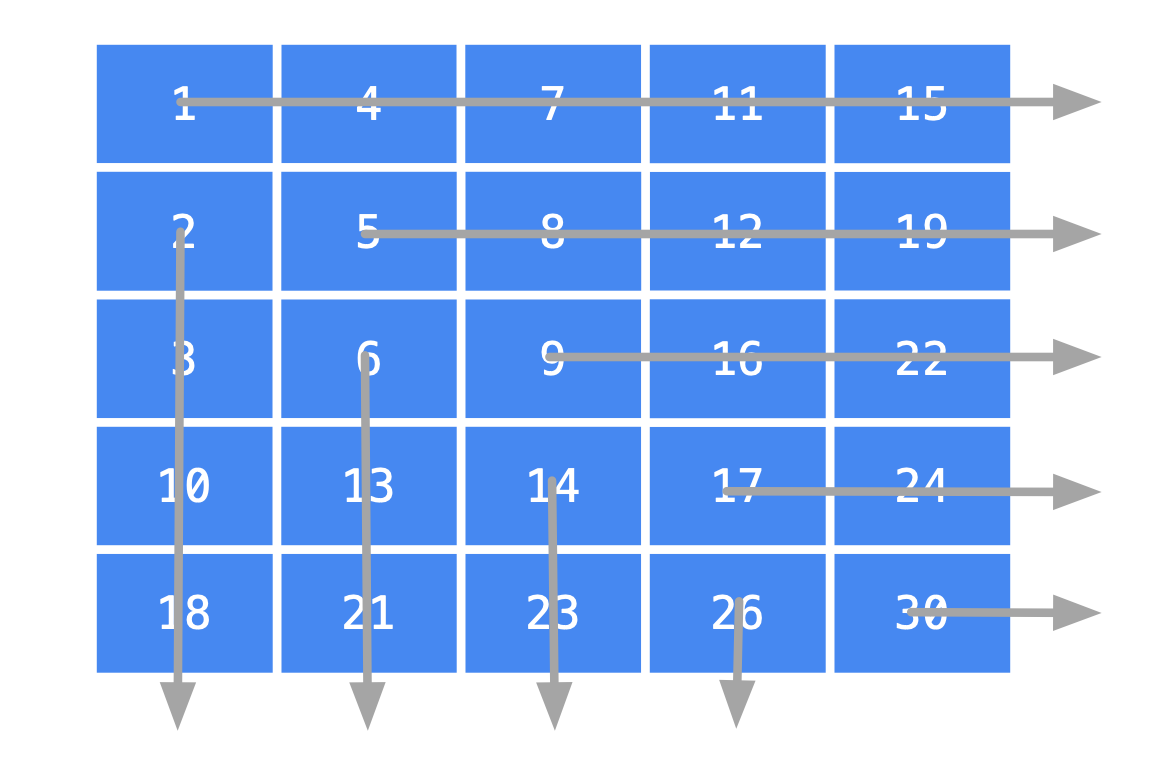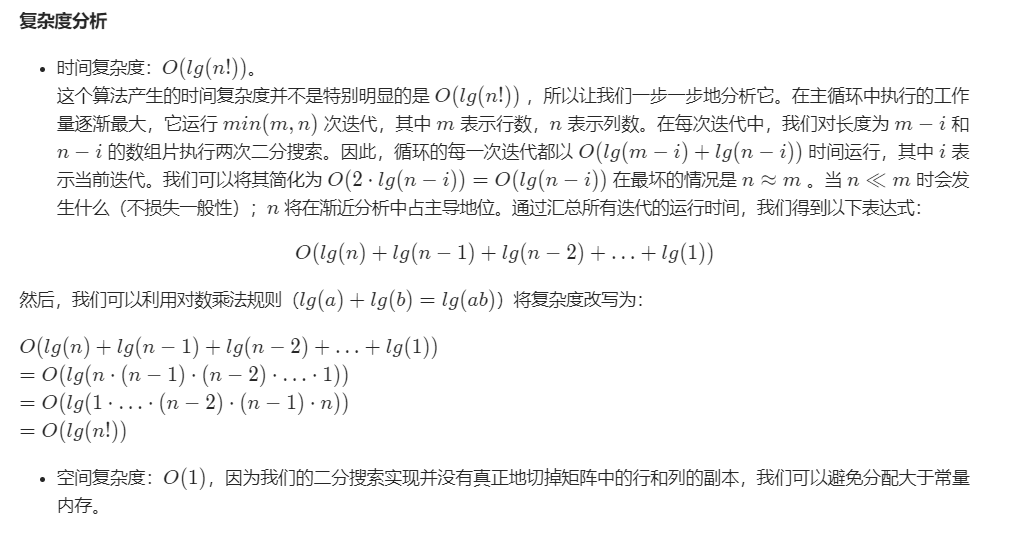# 【leetcode】240.搜索二维矩阵 II （贪心+动态规划，动图详解）

#### 240. 搜索二维矩阵 II

• 每行的元素从左到右升序排列。
• 每列的元素从上到下升序排列。

[
[1,   4,  7, 11, 15],
[2,   5,  8, 12, 19],
[3,   6,  9, 16, 22],
[10, 13, 14, 17, 24],
[18, 21, 23, 26, 30]
]


• 如果我们要用二分查找法，可以发现，如果一行的开头那个元素就比目标元素大，那么这一行的所有元素，以及行号大于这一行的元素都不在考虑的范围内。
• 我们首先尝试从左上角开始走，发现横着走数值增大，竖着走数值也增大，目标数值这在两个方向上都有可能存在。那如果我们从右上角或者左下角除法，找目标元素，那就不一样了，于是有了下面的“排除法”。

### 方法一：减而治之

• 1、如果选择左下角为起点，以下展示了“减治”的过程。public class Solution {

public boolean searchMatrix(int[][] matrix, int target) {
int rows = matrix.length;
if (rows == 0) {
return false;
}
int cols = matrix.length;
if (cols == 0) {
return false;
}

// 起点：左下角
int x = rows - 1;
int y = 0;
// 不越界的条件是：行大于等于 0，列小于等于 cols - 1
while (x >= 0 && y < cols) {
// 打开注释，可以用于调试的代码
// System.out.println("沿途走过的数字：" + matrix[x][y]);
if (matrix[x][y] > target) {
x--;
} else if (matrix[x][y] < target) {
y++;
} else {
return true;
}
}
return false;
}
}


• 时间复杂度：O(M + N)，M是这个矩阵的行数，N 是这个矩阵的列数，我们看到，这种算法是“不回头”的，至多走 M + N 步就能搜索到目标数值，或者判定目标数值在矩阵中不存子啊。

• 空间复杂度：O(1)，算法使用了常数个变量。

• 2、如果选择右上角为起点，以下展示了“减治”的过程。public class Solution {

public boolean searchMatrix(int[][] matrix, int target) {
// 特判
int rows = matrix.length;
if (rows == 0) {
return false;
}
int cols = matrix.length;
if (cols == 0) {
return false;
}

// 起点：右上角
int x = 0;
int y = cols - 1;

// 不越界的条件是：行小于等于 rows - 1，列大于等于 0
while (x < rows && y >= 0) {
// 打开注释，可以用于调试的代码
// System.out.println("沿途走过的数字：" + matrix[x][y]);
if (matrix[x][y] > target) {
y--;
} else if (matrix[x][y] < target) {
x++;
} else {
return true;
}
}
return false;
}
}


（同上）。

### 方法二：二分查找• Java
class Solution {
private boolean binarySearch(int[][] matrix, int target, int start, boolean vertical) {
int lo = start;
int hi = vertical ? matrix.length-1 : matrix.length-1;

while (hi >= lo) {
int mid = (lo + hi)/2;
if (vertical) { // searching a column
if (matrix[start][mid] < target) {
lo = mid + 1;
} else if (matrix[start][mid] > target) {
hi = mid - 1;
} else {
return true;
}
} else { // searching a row
if (matrix[mid][start] < target) {
lo = mid + 1;
} else if (matrix[mid][start] > target) {
hi = mid - 1;
} else {
return true;
}
}
}

return false;
}

public boolean searchMatrix(int[][] matrix, int target) {
// an empty matrix obviously does not contain target
if (matrix == null || matrix.length == 0) {
return false;
}

// iterate over matrix diagonals
int shorterDim = Math.min(matrix.length, matrix.length);
for (int i = 0; i < shorterDim; i++) {
boolean verticalFound = binarySearch(matrix, target, i, true);
boolean horizontalFound = binarySearch(matrix, target, i, false);
if (verticalFound || horizontalFound) {
return true;
}
}

return false;
}
}03-18127
04-0319602-27148
03-062503
10-0479
08-19157
03-311259
08-171万+
09-283266
06-14616